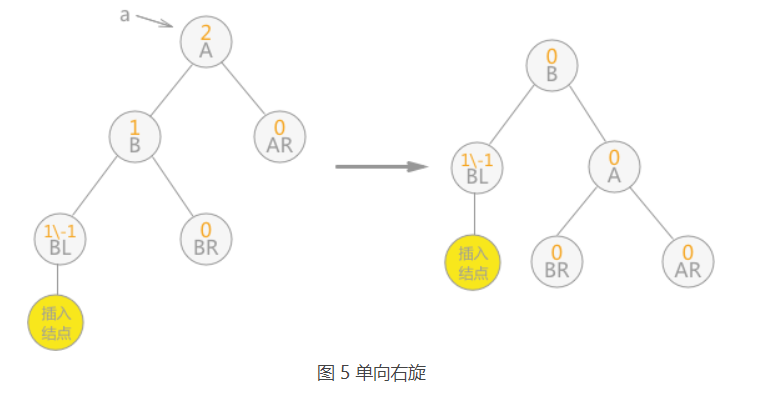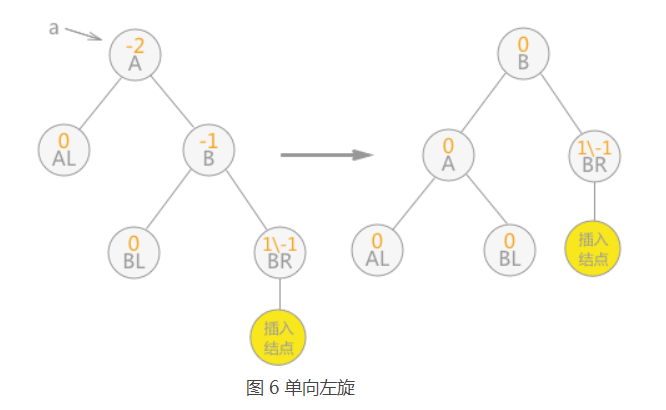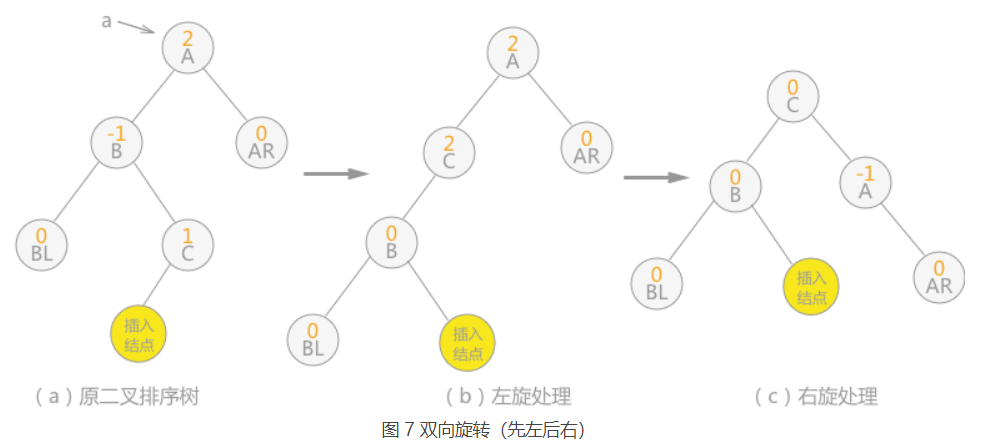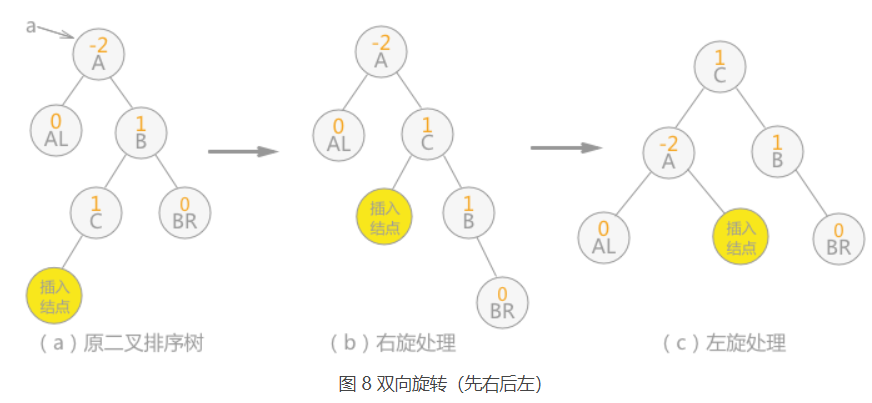# 数据结构查找算法之平衡二叉树【附Java代码实现，图解】

• 平衡二叉树，又称为 AVL 树。实际上就是遵循以下两个特点的二叉树：
• 每棵子树中的左子树和右子树的深度差不能超过 1；
• 二叉树中每棵子树都要求是平衡二叉树；
• 平衡因子：每个结点都有其各自的平衡因子，表示的就是其左子树深度同右子树深度的差。平衡二叉树中各结点平衡因子的取值只可能是：0、1 和 -1。
• 二叉排序树转化为平衡二叉树；当平衡二叉树由于新增数据元素导致整棵树的平衡遭到破坏时，就需要根据实际情况做出适当的调整，假设距离插入结点最近的“不平衡因子”为 a。则调整的规律可归纳为以下 4 种情况：
• 单向右旋平衡处理：若由于结点 a 的左子树为根结点的左子树上插入结点，导致结点 a 的平衡因子由 1 增至 2，致使以 a 为根结点的子树失去平衡，则只需进行一次向右的顺时针旋转，如图五；
• 单向左旋平衡处理：如果由于结点 a 的右子树为根结点的右子树上插入结点，导致结点 a 的平衡因子由 -1变为 -2，则以 a 为根结点的子树需要进行一次向左的逆时针旋转，如图六；
•  双向旋转（先左后右）平衡处理：如果由于结点 a 的左子树为根结点的右子树上插入结点，导致结点 a 平衡因子由 1 增至 2，致使以 a 为根结点的子树失去平衡，则需要进行两次旋转操作，如图七；
• 双向旋转（先右后左）平衡处理：如果由于结点 a 的右子树为根结点的左子树上插入结点，导致结点 a 平衡因子由 -1 变为 -2，致使以 a 为根结点的子树失去平衡，则需要进行两次旋转（先右旋后左旋）操作，如图八；
•class TreeNode {
int bf; //balance flag(平衡因子：表示左子树深度与右子树的差)
int val;
TreeNode left;
TreeNode right;
TreeNode(int x) { val = x; }
}

public class Main {
//对以 p 为根结点的二叉树做右旋处理，令 p 指针指向新的树根结点
public void rightRotate(TreeNode p){
//借助图 5 所示加以理解，其中结点 A 为 p 指针指向的根结点
TreeNode node = p.left;
p.left = node.right;
node.right = p;
p = node;
}

//对以 p 为根结点的二叉树做左旋处理，令 p 指针指向新的树根结点
public void leftRotate(TreeNode p){
//借助图 6 所示加以理解，其中结点 A 为 p 指针指向的根结点
TreeNode node = p.right;
p.right = node.left;
node.left = p;
p = node;
}

//对二叉树的左子树做平衡处理;结合图进行查看，bf代表平衡因子（表示左子树深度同右子树深度的差）与图中一致
public void leftBalance(TreeNode T){
TreeNode node = T.left;
TreeNode node1;
//查看以 T 的左子树为根结点的子树，失去平衡的原因，
//如果 bf 值为 1 ，则说明添加在左子树为根结点的左子树中，需要对其进行右旋处理；
//反之，如果 bf 值为 -1，说明添加在以左子树为根结点的右子树中，需要进行双向先左旋后右旋的处理
switch(node.bf){
case 1:
T.bf = 0;
node.bf = 0;
rightRotate(T);
break;
case -1:
node1 = node.right;
switch (node1.bf){
case 1:
T.bf = -1;
node.bf = 0;
break;
case 0:
T.bf = 0;
node.bf = 0;
break;
case -1:
T.bf = 0;
node.bf = 1;
break;
}
node1.bf = 0;
leftRotate(T.left);
rightRotate(T);
break;
}
}

//右子树的平衡处理同左子树的平衡处理完全类似
public void rightBalance(TreeNode T){
TreeNode node = T.right;
TreeNode node1;
switch(node.bf){
case -1:
T.bf = 0;
node.bf = 0;
leftRotate(T);
break;
case 1:
node1 = node.left;
switch (node1.bf){
case 1:
T.bf = 0;
node.bf = -1;
break;
case 0:
T.bf = 0;
node.bf = 0;
break;
case -1:
T.bf = 0;
node.bf = 1;
break;
}
node1.bf = 0;
rightRotate(T.right);
leftRotate(T);
break;
}
}

/**
* 二叉平衡树插入元素
* @param T 要插入元素的二叉平衡树
* @param e 要插入的元素
* @param taller 插入元素之后树的高度是否增加
* @return
*/
public int insertAVL(TreeNode T, int e, boolean taller){
//如果本身为空树，则直接添加 e 为根结点
if(T == null){
T.bf = 0;
T.val = e;
taller = true;
}else if(e == T.val){    //如果二叉排序树中已经存在 e ，则不做任何处理
taller = false;
return 0;
}else if(e < T.val){     //如果 e 小于结点 T 的数据域，则插入到 T 的左子树中
//如果插入过程，不会影响树本身的平衡，则直接结束
if(insertAVL(T.left, e, taller) == 0)
return 0;
//判断插入过程是否会导致整棵树的深度 +1
if(taller){
//判断根结点 T 的平衡因子是多少，由于是在其左子树添加新结点的过程中导致失去平衡，
// 所以当 T 结点的平衡因子本身为 1 时，需要进行左子树的平衡处理，否则更新树中各结点的平衡因子数
switch (T.bf){
case 1:
leftBalance(T);
taller = false;
break;
case 0:
T.bf = 1;
taller = true;
break;
case -1:
T.bf = 0;
taller = false;
break;
}
}
}else if(e > T.val){     //如果 e 大于结点 T 的数据域，则插入到 T 的右子树中
if(insertAVL(T.right, e, taller) == 0)
return 0;
if(taller){
switch (T.bf){
case 1:
T.bf = 0;
taller = false;
break;
case 0:
T.bf = -1;
taller = true;
break;
case -1:
rightBalance(T);
taller = false;
break;
}
}
}
return 1;
}
}

07-229482

#### 图示讲解AVL平衡二叉树的左旋和右旋

10-051万+

#### 二叉树的左旋和右旋04-2776

#### AVL平衡二叉树原理解析全网最详细，最清晰解释欢迎相互学习 新人访问少，但绝对是干货

02-28123

#### 二叉树的左右旋转

01-0779

#### 平衡二叉树（AVL树）及C语言实现©️2020 CSDN 皮肤主题: 大白 设计师: CSDN官方博客点击重新获取扫码支付1.余额是钱包充值的虚拟货币，按照1:1的比例进行支付金额的抵扣。
2.余额无法直接购买下载，可以购买VIP、C币套餐、付费专栏及课程。余额充值